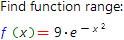# Function range online calculatorThe range of some function f(x) is a set, containing all the values that can be obtained by substituting into this function all the valid values of the argument x. The range of the function is denoted by E(f).

Illustrate the above with a concrete example. Consider the function f(x) = e−x2, which graph is shown on the figure.Its easy to mention, that whatever values of argument x we would not substitute into the function f(x), the returning value will always be in the range from 0 to 1. Thus, the range of the function in question is from 0 to 1.

This fact can be written as follows:

E(f)(0; 1]

Our online calculator is based on Wolfram Alpha system. The calculator allows to find the range of almost any function.

Function range calculatorYou want to find function range